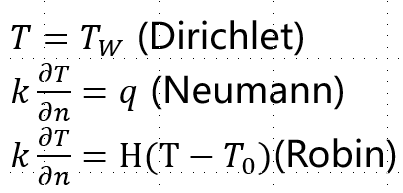# The parameters of wall heating model

Hi, everyone! I found that there are three types of wall heat condition in MFIX BC, they are constant wall temperature, constant heat flux and transfer coefficient, and I wonder know does the three types means the three types of boundary condition?and I found that the unit of constant heat flux is (K/m), does it calculated by q/k(q-heat flux unit:W/m2; k-heat transfer coefficient unit:W/km),and the unit of transfer coefficient is (/m), does it calculated by h/k(h-conductivity unit:W/Km2, k-heat transfer coefficient unit:W/k*m)

I encountered a similar problem in TFM. I test the the Hw(/m)=Hw(w/m2k)/kg,but the result is far from the experimental results. I dont know why.